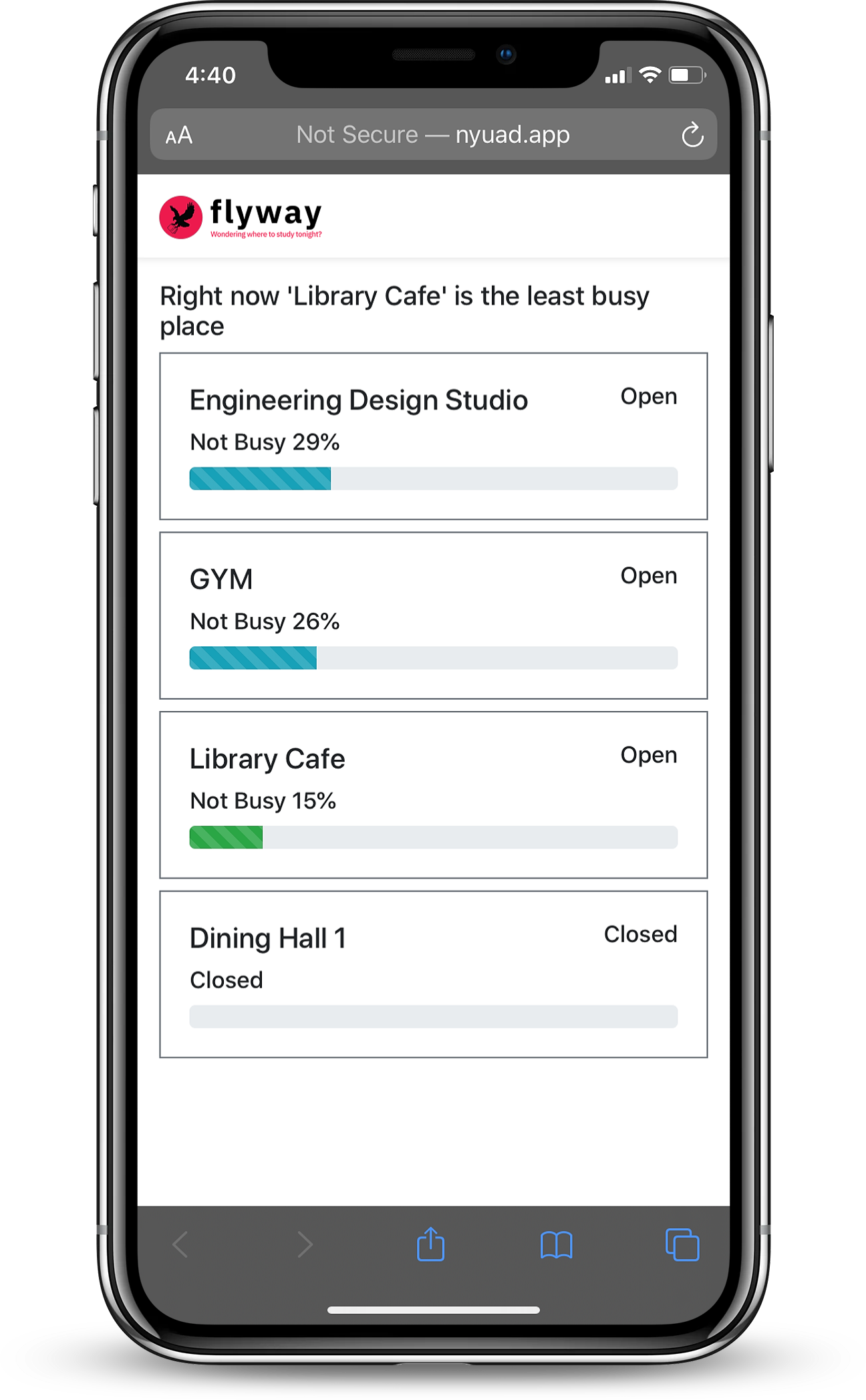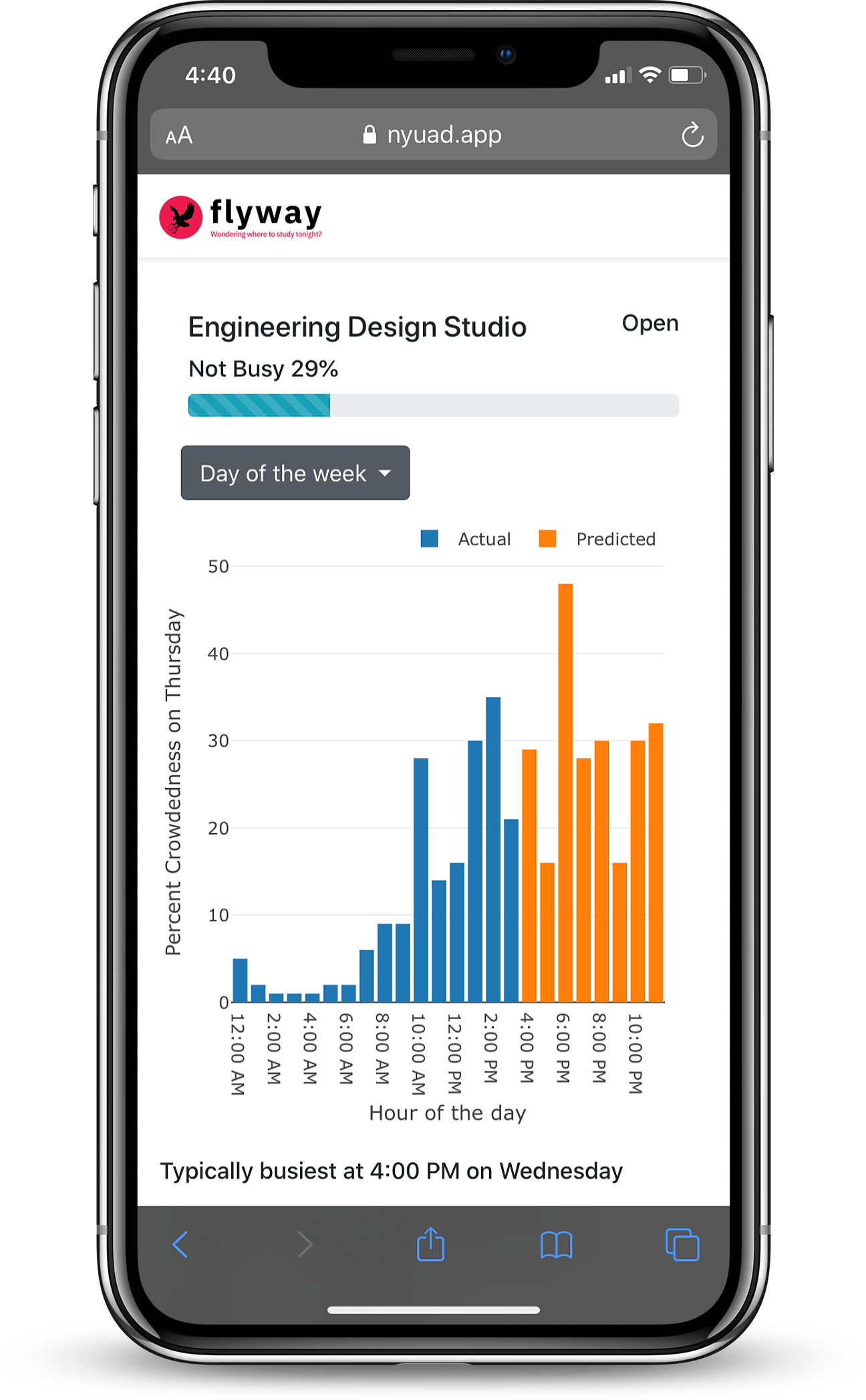# flyway: crowdedness forecasting with LSTM

flyway was a machine learning project to explore the LSTM RNN as a tool for forecasting crowdedness in the Engineering Design Studio (EDS) at NYUAD. A project by @niniack & @woswos

“Every once in a while, you may be faced with the dreaded decision of where to study. We use past network traffic data to predict how crowded a space might be, whether this means high traffic, for those who enjoy company, or low traffic, for those who want quiet. Either way, you’ll know before anyone knows.”

Check out the notebook (Github)

Check out the final submission (PDF)## Data Collection

To begin with, we figured that it would be worth looking at network traffic data from personal devices and extract some sort of information from there to use as a crowdedness metric. We also figured that without any data correlating network traffic to a headcount, it would have been silly for us to predict the number of people, as we would have no “target data”, so to speak.

So, we set up a computer in the EDS with the wifi chip set to monitoring mode, collecting the network traffic in its vicinity. To do this, we made use of the `dumpcap` tool, which provides users with the `-I` flag to enter `--monitor-mode`.

Having collected over a million data packets, we exported the raw data as a CSV file to work with in a python notebook.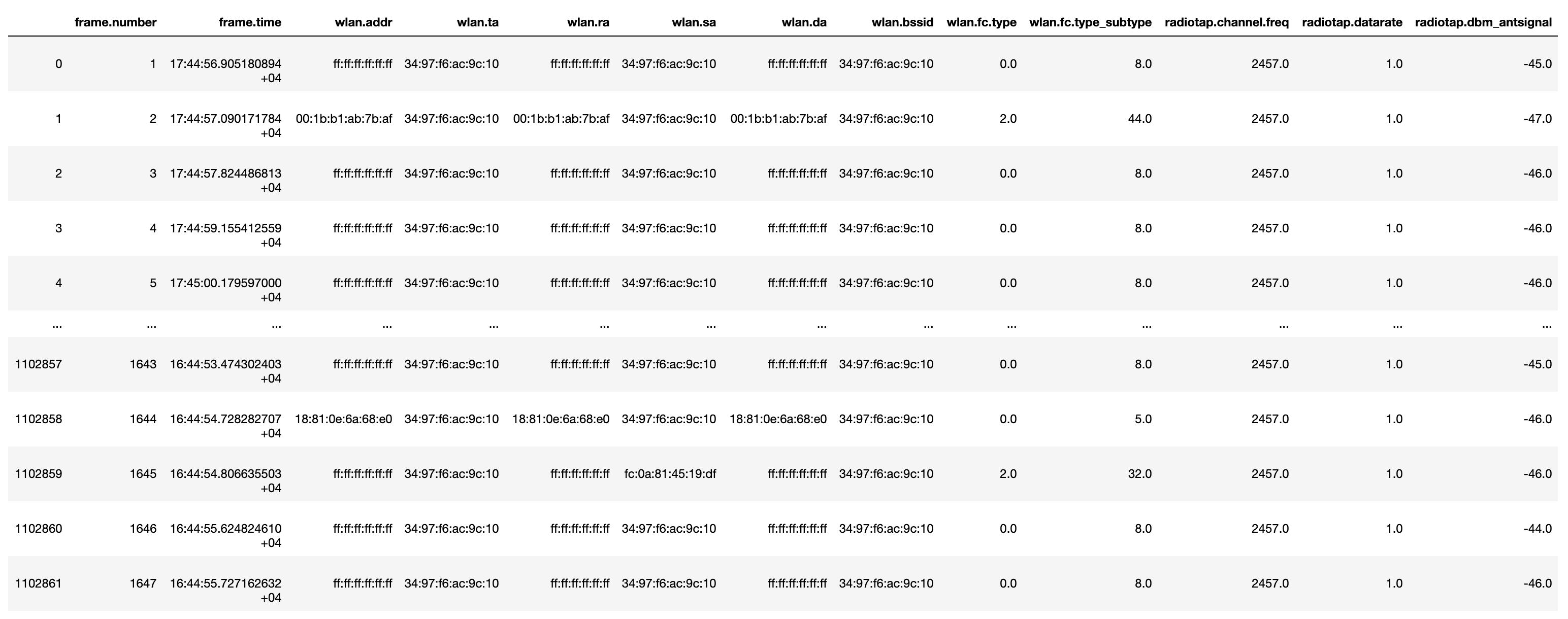Raw data collected in the EDS

## Data Visualization

Looking at the dataset, we decided to plot the number of frames sent by hour. This could provide a rough estimate for how the space looked at certain times of day (hour of day) and certain days (day of week). More network traffic likely implied more people.

Cleaning the data proved to be an important step. As the EDS is home to a number of non-human devices (RPis from student projects, desktops, etc), we wanted to remove this traffic from the dataset. To do this, we obtained the list of these devices and removed them from our dataset. We also removed the top 10 “loudest” devices, as these most likely included routers and other static devices not part of the EDS network (e.g. nearby cameras). The two graphs below show the drastic difference as a result of data cleaning.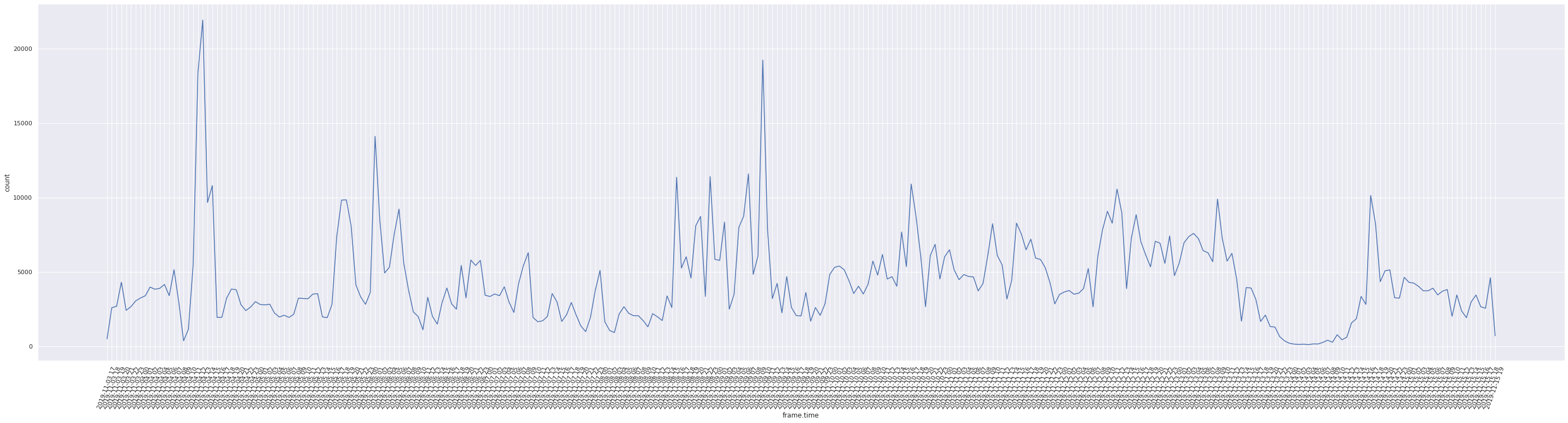Frame count plotted by hour prior to cleaning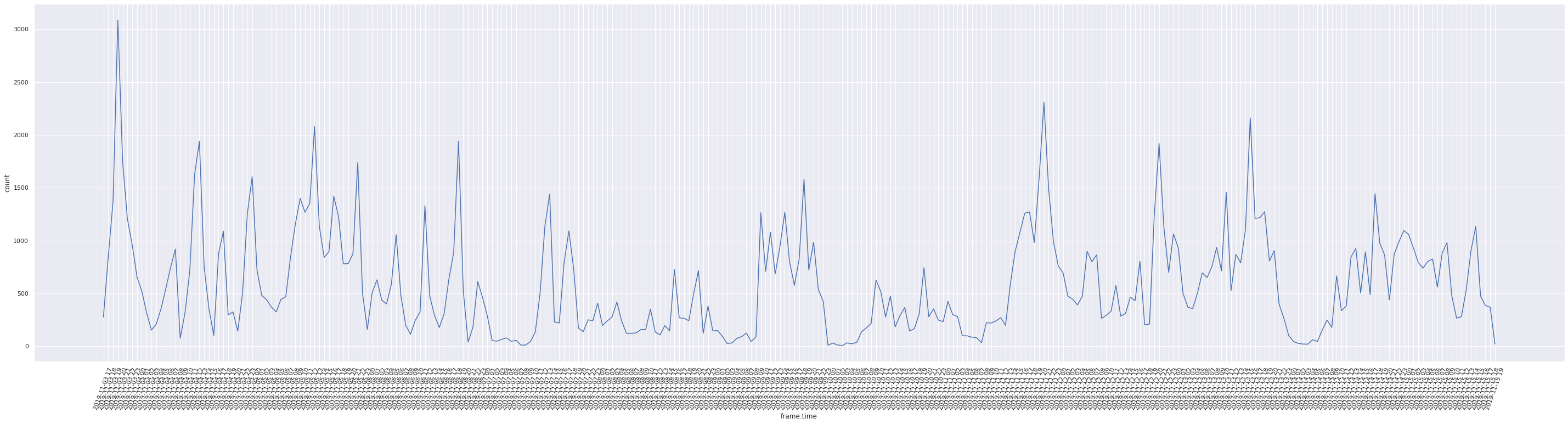Frame count plotted by hour after cleaning

It is also worth noting that there is a relatively large discrepancy in frame count trend on the first hour of the first day and the last hour of the last day as data collection was not run for the full hour. This could have seriously affected our model evaluation and so the relevant values were removed.

We also plot the frame count and unique MAC addresses per hour, normalized, to better understand if they are a good representation of crowdedness.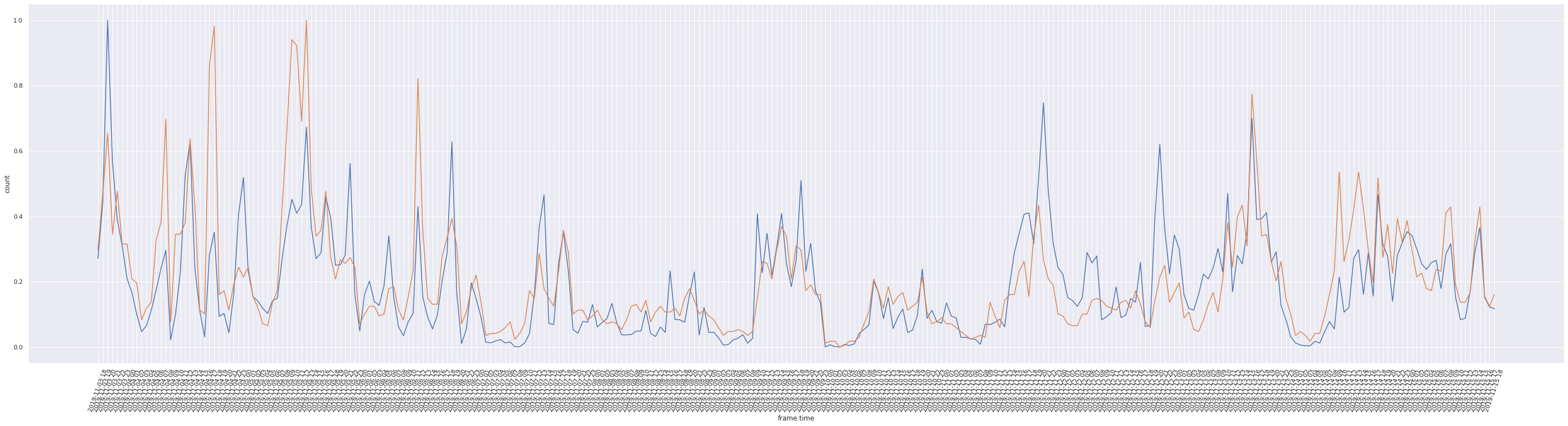Frame count and unique MAC count per hour normalized

While there are a few anomalies, the graph above shows that the two metrics act as a pretty estimator for how busy the area is. An example of an anomaly may be the case where there is a large number of unique MAC addresses but relatively few packages being sent. This could represent a situation where a group of people walked into the space but ultimately decided to not stay. To hedge against this, we can use a weighted average that favors the lower value, always treating the larger metric as an anomaly:

``````def weighted_average(val1, val2):
"""
Calculate weighted average, giving weight to the smaller value
"""

weight = 0.8

if (val1 > val2):
weighted_avg = (weight*val2 + (1-weight)*val1)
else:
weighted_avg = (weight*val1 + (1-weight)*val2)

return weighted_avg
``````

Applying this technique produces the following graph: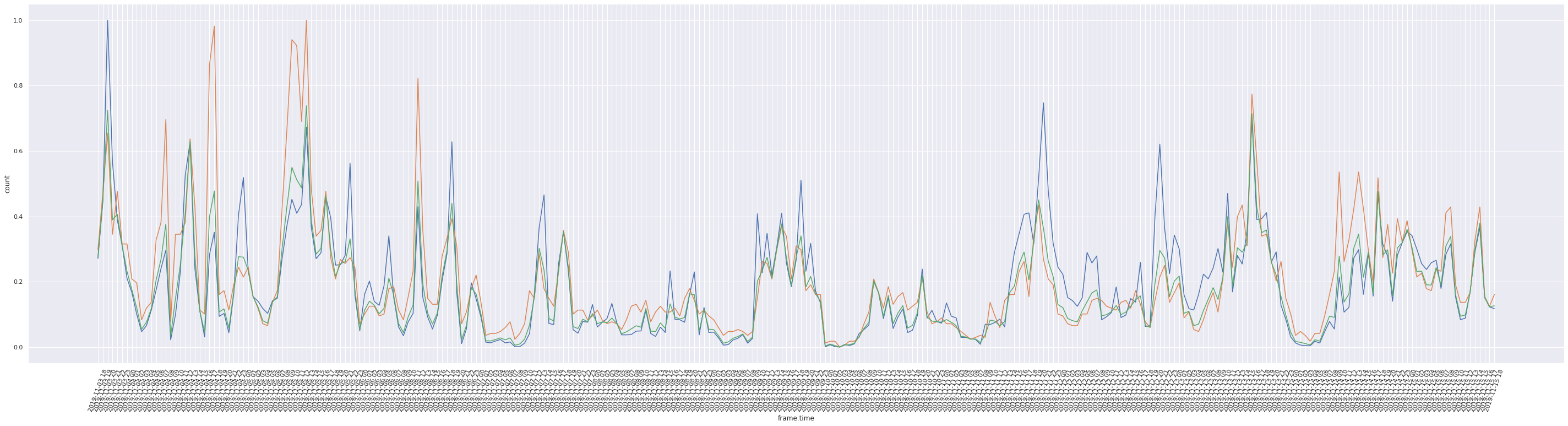Frame count, unique MAC count, and crowdedness per hour normalized

## Data Engineering and Consideration

So far the model would only have information about the past frame data. We can supplement the model by adding more features:

• Weekday/Weekend
• Hour of the Day
• Day of the Week

We may also improve the accuracy of our model if we input scaled data into the model as well as one hot encode all categorical values.

We also carried out this process for the number of unique MAC addresses per hour, resulting in the two metrics we planned to use for busyness.

## Data Preparation

So far, we have three datasets to work with:

• Frame count per hour
• Unique MAC count per hour
• Biased weighted average of frame & unique MAC count normalized

As we fed frame count and unique MAC count into the model as inputs, and the plan was to use LSTM cells, we reshaped our data following this tutorial. Next, we split each of these datasets into training and testing (70-30 split)

``````def split_dataframe(data, n_in=1, n_out=1, dropnan=True):

"""
Split a dataframe for LSTM input
"""

n_vars = 1 if type(data) is list else data.shape
# df = pd.DataFrame(data)
df = data
cols, names = list(), list()

# input sequence (t-n, ... t-1)
for i in range(n_in, 0, -1):
cols.append(df.shift(i))
names += [('%s(t-%d)' % (df.columns[j], i)) for j in range(n_vars)]

# forecast sequence (t, t+1, ... t+n)
for i in range(0, n_out):
cols.append(df.shift(-i))
if i == 0:
names += [('%s(t)' % (df.columns[j])) for j in range(n_vars)]
else:
names += [('%s(t+%d)' % (df.columns[j], i)) for j in range(n_vars)]

# put it all together
agg = pd.concat(cols, axis=1)
agg.columns = names

# drop rows with NaN values
if dropnan:
agg.dropna(inplace=True)

return agg
``````

Function from machinelearningmastery

## Model Design

Having reshaped and split our dataframes, we make attempts at building a reasonable model. Our goal is to feed in the frame and umac count `t-n to t` and produce a crowdedness measure at `t+1`.

### Model 1

We make a naive attempt, simply feeding in the data into stacked LSTM cells, concatenate the outputs, and feed the merged data into a dense network.

``````##  define input
visible1 = Input(shape=(n_steps_fc, n_features_fc))
##  first interpretation model
hidden1 = LSTM(250, activation='relu', return_sequences=True, dropout=0.12)(visible1)
hidden2 = LSTM(250, activation='relu', return_sequences=True)(hidden1)
hidden3 = LSTM(250, activation='relu')(hidden2)
frame_count = Dense(1)(hidden3)
##  define output
frame_count_model = Model(visible1, frame_count)

##  define input
visible2 = Input(shape=(n_steps_umac, n_features_umac))
##  second interpretation  model
hidden4 = LSTM(200, activation='relu', return_sequences=True, dropout=0.2)(visible2)
hidden5 = LSTM(200, activation='relu', return_sequences=True)(hidden4)
hidden6 = LSTM(200, activation='relu')(hidden5)
umac_count = Dense(1)(hidden6)
umac_count_model = Model(visible2, umac_count)

merge = concatenate([frame_count, umac_count])
hidden7 = Dense(512)(merge)
hidden8 = Dense(256)(hidden7)
hidden9 = Dense(256)(hidden8)
busyness = Dense(1)(hidden9)

model = Model(inputs=[visible1, visible2], outputs=busyness)
print(model.summary())
``````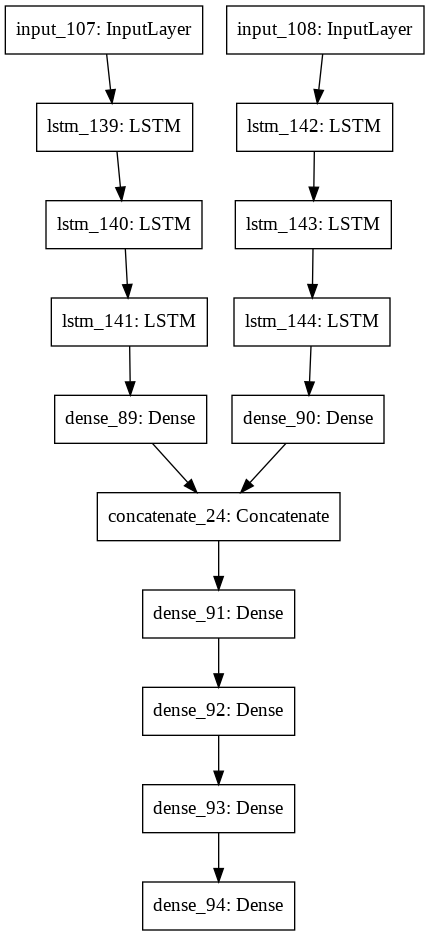However, the issue here is that the model is a bit of a blackbox. Turning parameters is a little overwhelming, considering there are 11 layers to play around with. We could solve this issue if we used a modularized approach!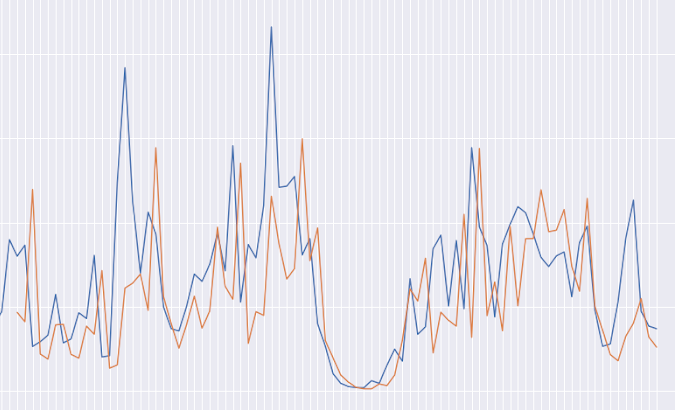Blue is the original data; orange is the forecasted data from model 1. Not that great!

### Model 2

Splitting the previous model into smaller components, results in this overview:Higher level overview of model 2

Taking a deeper look, we see how it was constructed:

``````##  encapsulating model
frames = Input(shape=(n_steps_fc, n_features_fc))
umacs = Input(shape=(n_steps_umac, n_features_umac))

out_frame = fc_model(frames)
out_umac = umac_model(umacs)

fc_model.trainable = False
umac_model.trainable = False

out_busyness = busyness_model([out_frame, out_umac])
busyness_model.trainable = False

overview_model = Model(inputs=[frames, umacs], outputs=out_busyness)
print(overview_model.summary())
``````

Each of the models within was pre-constructed and separately trained. The `fc-model` was given `t-3 to t` frame count data to predict the frame count at `t+1`. Similarly, the `umac-model` was given `t-3 to t` unique MAC counts to produce a count at `t+1`. Finally, the `busyness-model` was provided with an `x` number of frame and unique MAC counts, along with a `x` number of crowdedness measures to model the relationship we wrote in the `weighted_average` function (see above).

Here’s what the model looks like in detail: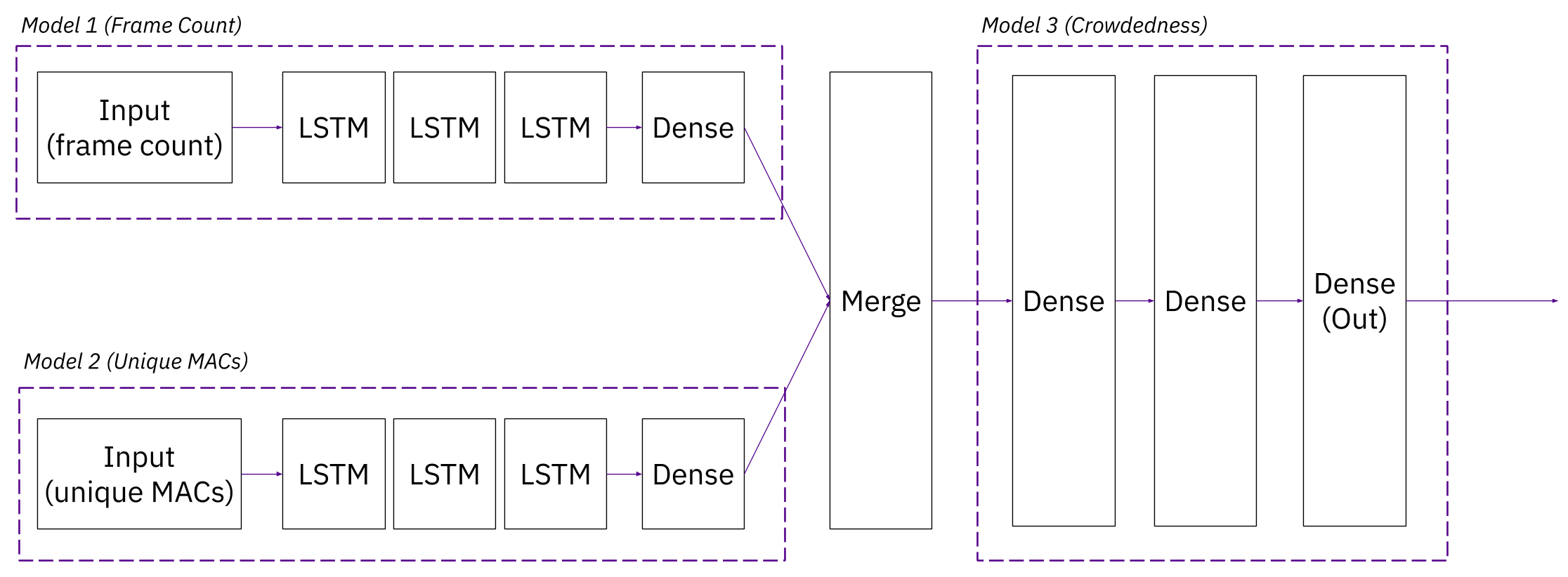Detailed overview of model 2

## Final Results

The second model performed well and allowed for much more control over tuning the parameters, as the train and test mean squared error (MSE) could be obtained for each of the three models within.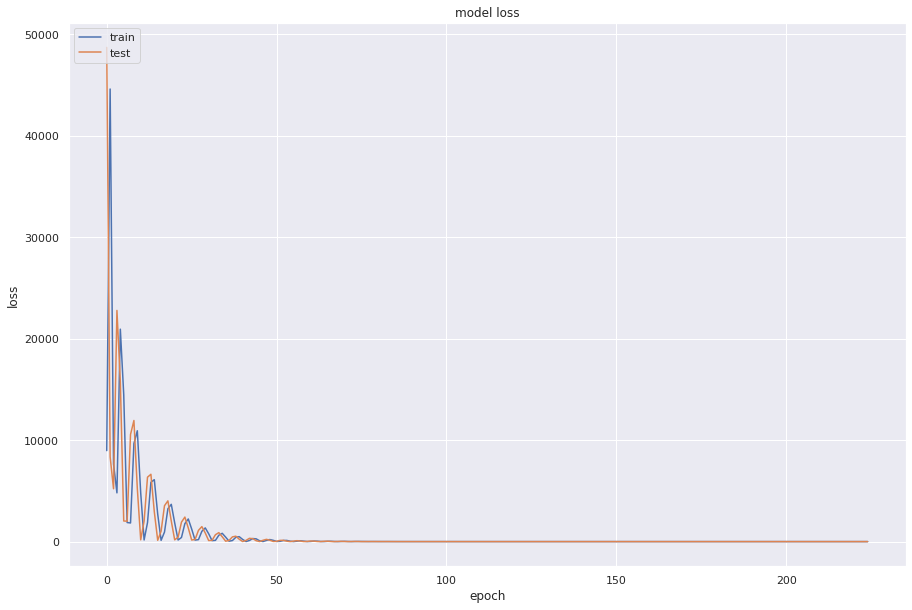Left to right: train/test MSE for fc_model, umac_model, busyness_model

The overall model had a train MSE of 2% and a test MSE of 1.3%

Finally, the graph: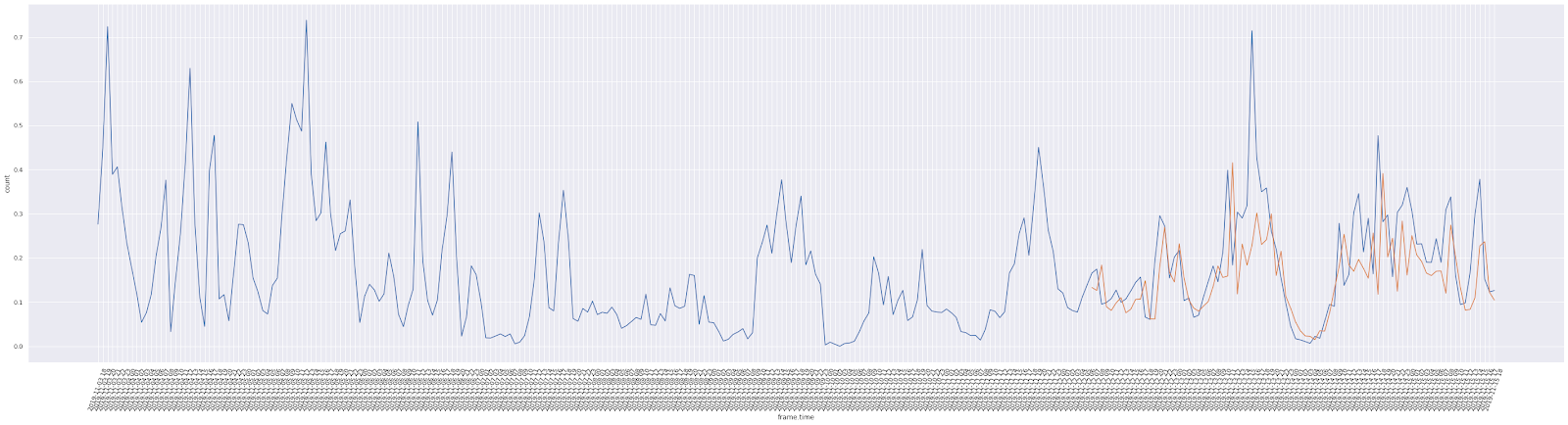We even created a UI to better represent our project results! Check it out at nyuad.app/flyway